Ex 9.3

Chapter 9 Class 11 Sequences and Series
Serial order wise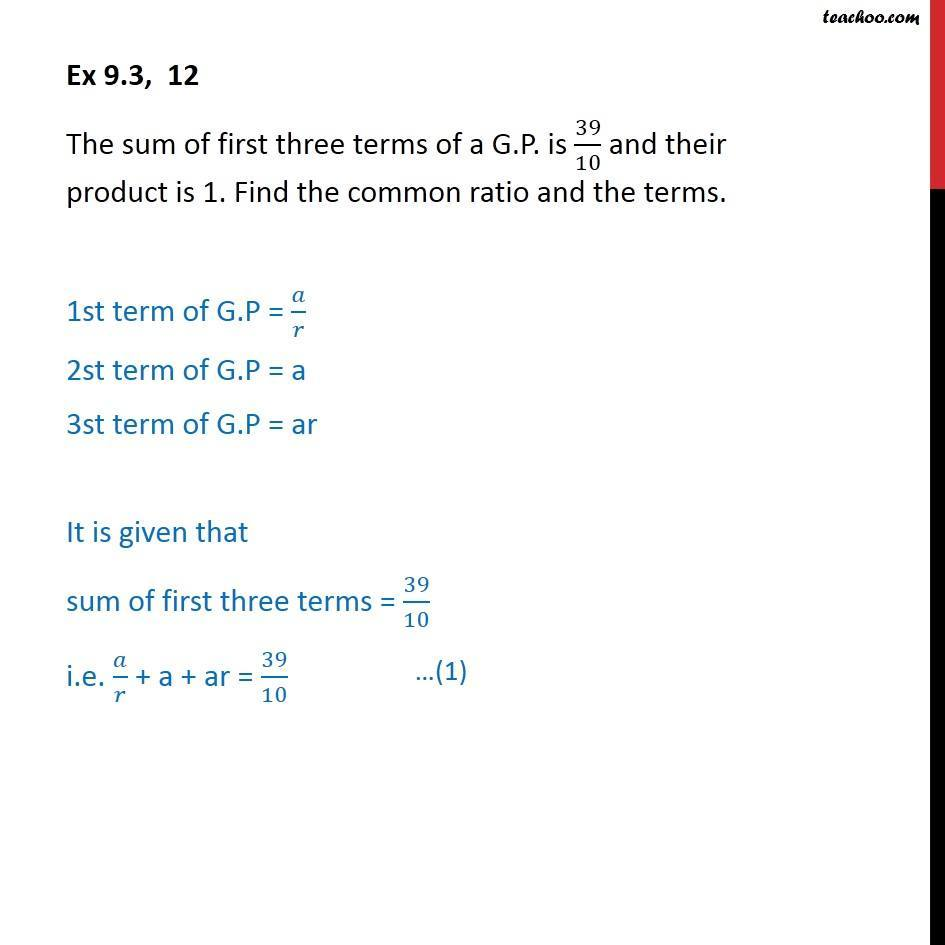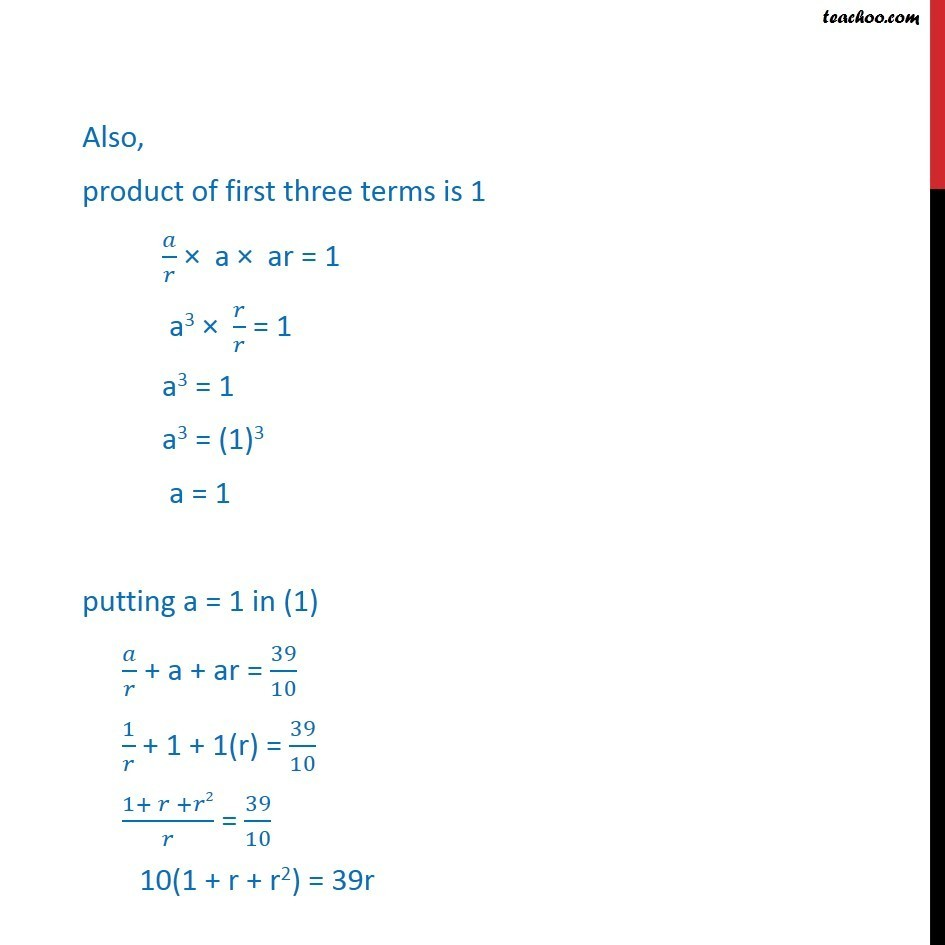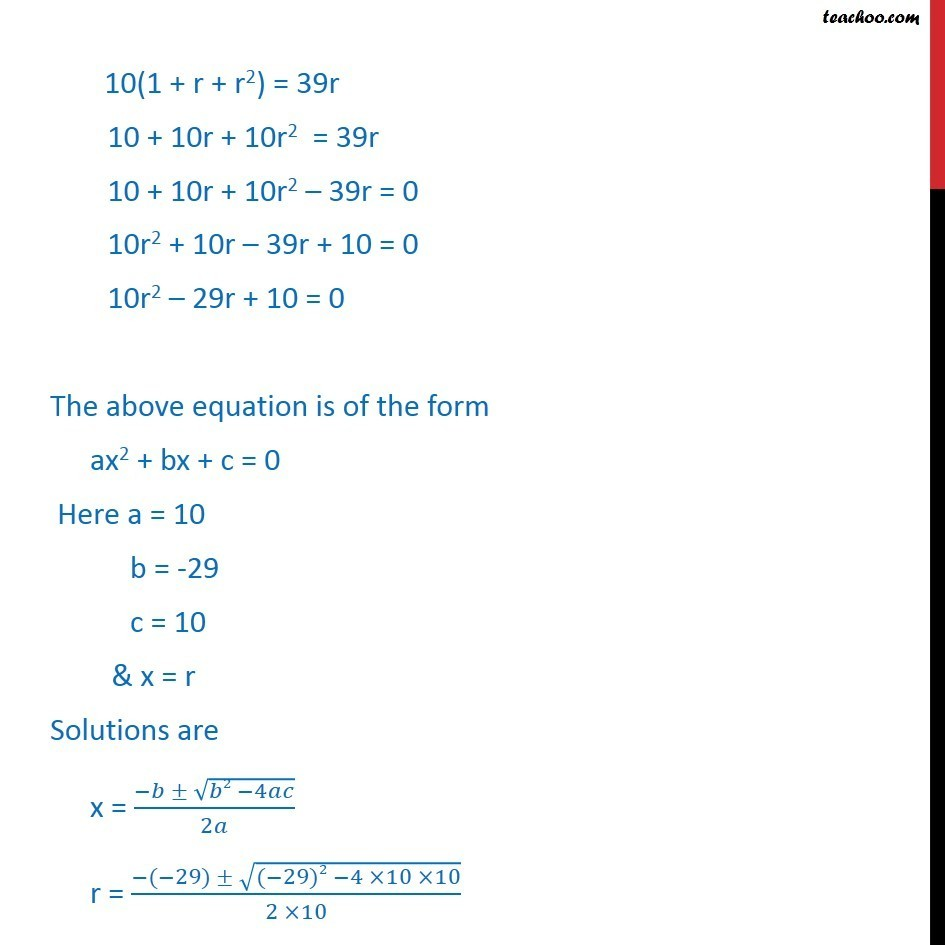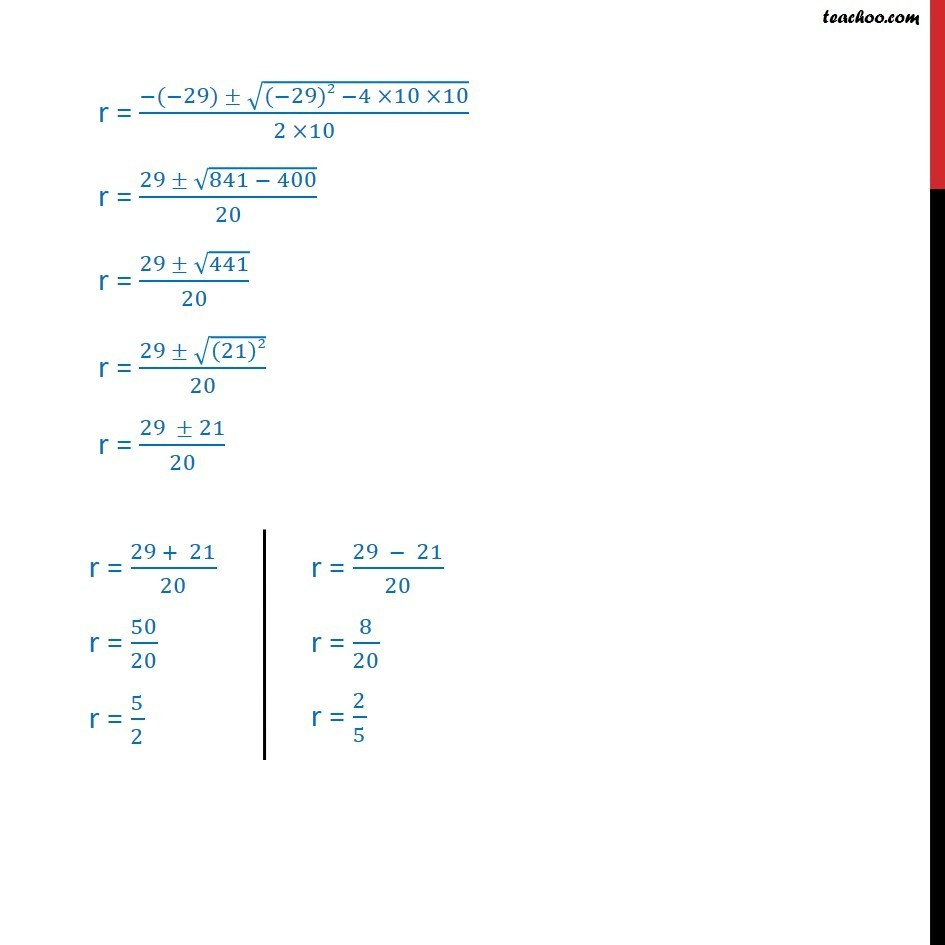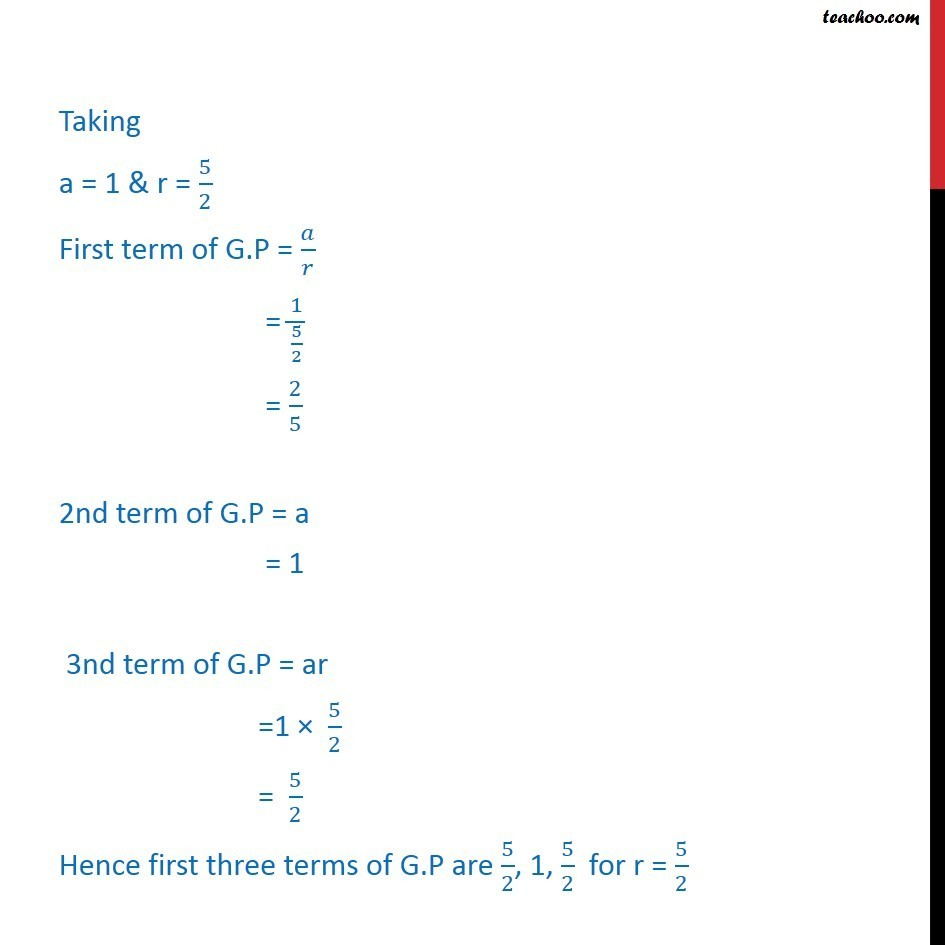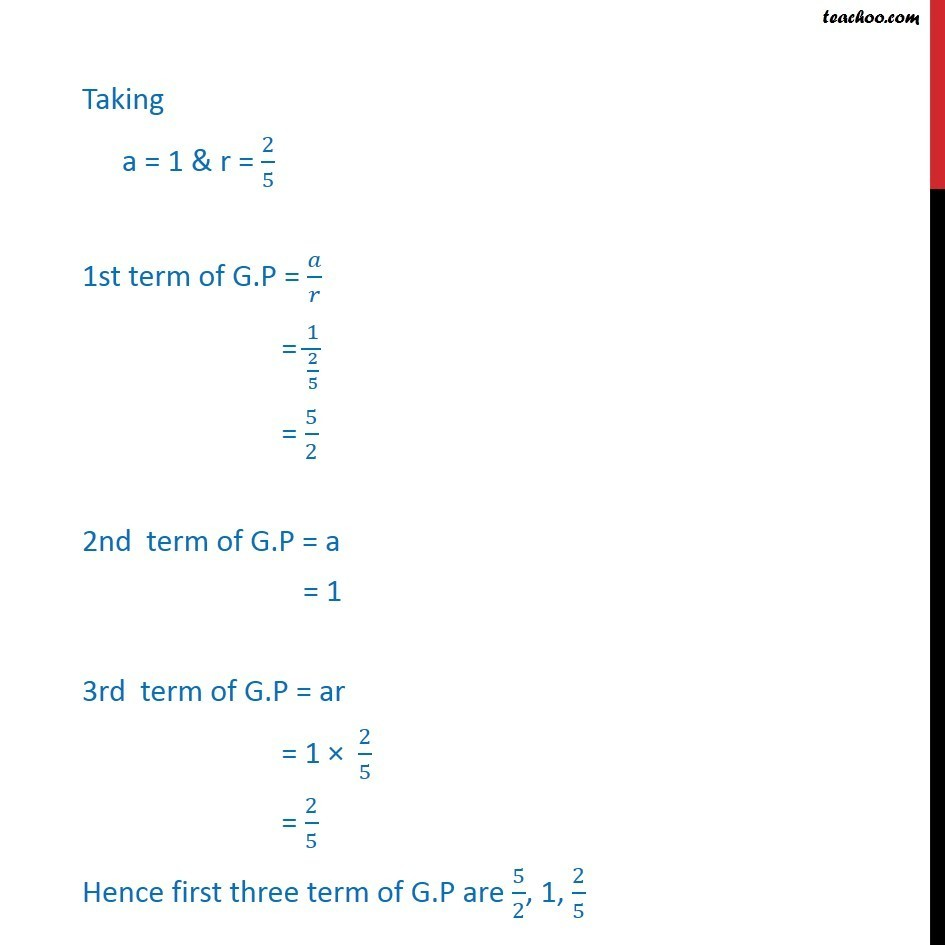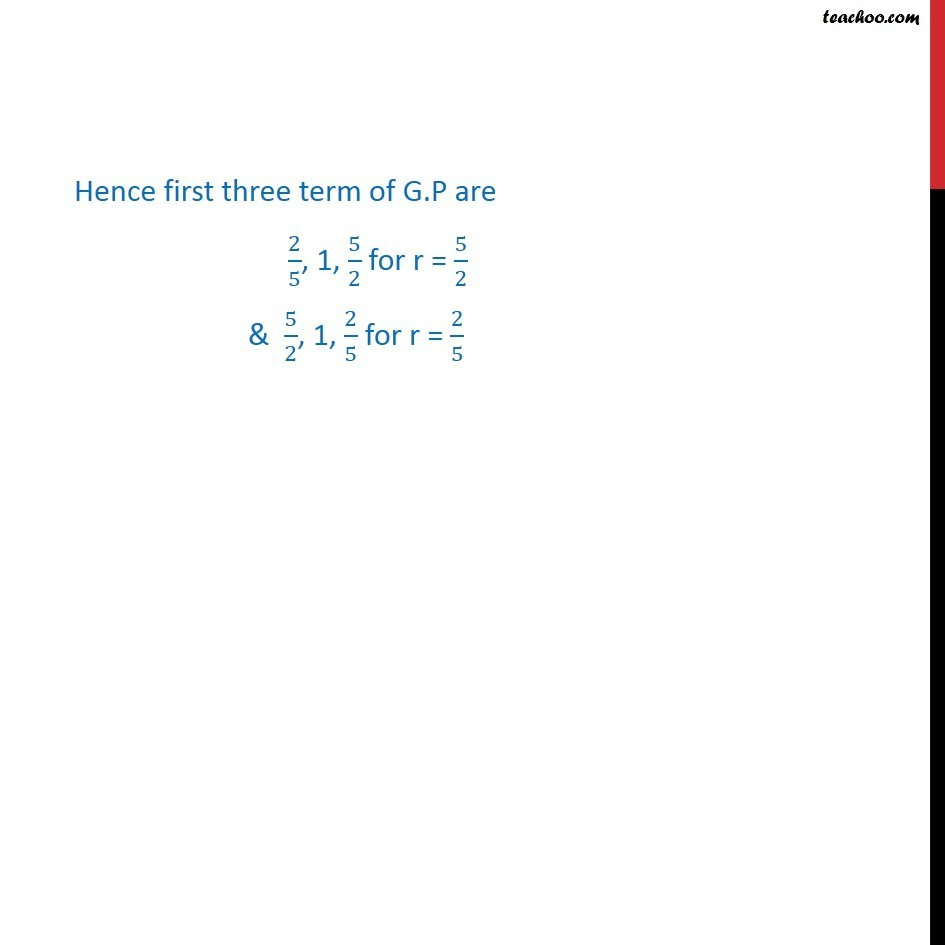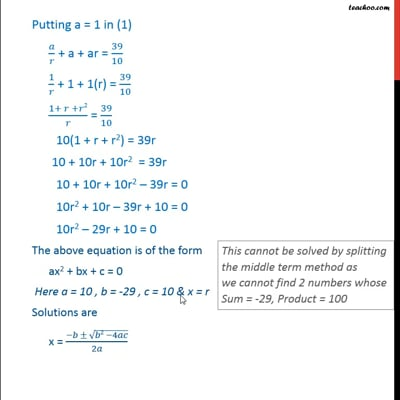This video is only available for Teachoo black users

Solve all your doubts with Teachoo Black (new monthly pack available now!)

### Transcript

Ex 9.3, 12 The sum of first three terms of a G.P. is 39/10 and their product is 1. Find the common ratio and the terms. 1st term of G.P = / 2st term of G.P = a 3st term of G.P = ar It is given that sum of first three terms = 39/10 i.e. / + a + ar = 39/10 Also, product of first three terms is 1 / a ar = 1 a3 / = 1 a3 = 1 a3 = (1)3 a = 1 putting a = 1 in (1) / + a + ar = 39/10 1/ + 1 + 1(r) = 39/10 (1+ + 2)/ = 39/10 10(1 + r + r2) = 39r 10(1 + r + r2) = 39r 10 + 10r + 10r2 = 39r 10 + 10r + 10r2 39r = 0 10r2 + 10r 39r + 10 = 0 10r2 29r + 10 = 0 The above equation is of the form ax2 + bx + c = 0 Here a = 10 b = -29 c = 10 & x = r Solutions are x = ( ( 2 4 ))/2 r = ( ( 29) (( 29)2 4 10 10))/(2 10) r = ( ( 29) (( 29)2 4 10 10))/(2 10) r = (29 (841 400))/20 r = (29 441)/20 r = (29 ((21)2))/20 r = (29 21)/20 Taking a = 1 & r = 5/2 First term of G.P = / = 1/(5/2) = 2/5 2nd term of G.P = a = 1 3nd term of G.P = ar =1 5/2 = 5/2 Hence first three terms of G.P are 5/2, 1, 5/2 for r = 5/2 Taking a = 1 & r = 2/5 1st term of G.P = / = 1/(2/5) = 5/2 2nd term of G.P = a = 1 3rd term of G.P = ar = 1 2/5 = 2/5 Hence first three term of G.P are 5/2, 1, 2/5 Hence first three term of G.P are 2/5, 1, 5/2 for r = 5/2 & 5/2, 1, 2/5 for r = 2/5